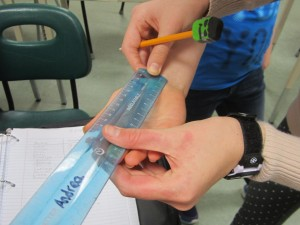# Jayden's Blog

## Artisticly Amazing!# Length of classmates hands!Hi everyone,

Do you know how long your hand is?

Our class has been learning about data sets, mean, median, mode and range. We did experiments to practice and learn about data analysis.

#### Our Inquiry question:

How long is your hand from the wrist to the tip of your finger.

#### Equipment:

Our group didn’t need much except for a ruler and peoples hands!

#### Procedure:

We would take people’s hand and measure from the wrist to the fingertip with a ruler.

#### Control standards:

We needed to make sure that We always measured from wrist to fingertip, always used cm on a clear ruler and if it was not on a exacted number than we round to the closest cm.

#### Predictions:

I predicted that the average length will be 16 Cm.

#### Our data:

Then we did the experiment and here is our data organized.

15,15,1516,16,16,16,16,16,16,17,17,17,17,17,17,17,18,18,20

#### Mean: 16.6

(how to calculate) 15+15+15+16+16+16+16+16+16+16+17+17+17+17+17+17+17+18+18+20÷20=16.6

#### Median: 16.5

(how to calculate) 16+17=33÷2=16.5

#### Mode: 16, 17

(how to calculate) Take the most common number, there can be more than one.

#### Range: 5

(how to calculate) largest number 20 subtract smallest number 15 is 5

The mode would be the best average because it is a size. (size of hand)

#### Conclusion:

I was kinda right with my prediction because  I predicted that 16 would be the average and I was right but 17 was also an average. Do you know what the length of your hand is? Try measuring it right now!

#### Extension:

If we were to extend this experiment I would sort it in to boy and girl to see if girls or boys had bigger hands.

Bye and have a cake-filled day

Jayden

Edited by Kaiya.

1.•